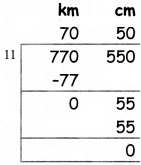Students can download 5th Maths Term 1 Chapter 4 Measurements InText Questions and Answers, Notes, Samacheer Kalvi 5th Maths Guide Pdf helps you to revise the complete Tamilnadu State Board New Syllabus, helps students complete homework assignments and to score high marks in board exams.

## Tamilnadu Samacheer Kalvi 5th Maths Solutions Term 1 Chapter 4 Measurements InText Questions

Try These (Text Book Page No. 65)

Convert into millimeters

Question 1.
90 cm
90 cm = 90 × 10
= 900 mm

Question 2.
5 cm 8 mm
5 cm 8 mm = 5 × 10 + 8 mm
= 50 + 8 = 58 mmQuestion 3.
5 m 9 mm
5 m 9 mm = 5 × 1000 + 9 mm
= 5009 mm

Try These (Text Book Page No. 66)

Convert into centimeter

Question 1.
8 m
8 m = 8 × 100
= 800 cm

Question 2.
6 m 4 cm
6m4cm = 6 × 10 + 4 = 600 + 4
= 604 cm

Question 3.
80 mm
80mm = 80 × 10
= 8 cmTry These (Text Book Page No. 66)

Convert into meter

Question 1.
8 km 400 m
8 km 400 m = (8 × 1000) + 400 m = 8000 + 400 = 8400 m

Question 2.
900 cm
900 cm = 900 + 100 = 9 m

Question 3.
3500 mm
3500 mm = 3500 + 1000 = 3 m 500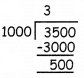Try These (Text Book Page No. 67)Convert into kilometers.

Question 1.
5430 m
5430 m = 5430 + 1000 = 5 km 430 mQuestion 2.
7500 m
7500 m = 7500 + 1000 = 7 km 500 m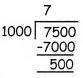Question 3.
8000 m
8000 m = 8000 + 1000 = 8 kmTry These (Text Book Page No. 68)

Subtract the following

Question 1.
1075 km 400 m – 27 km 350 m
Difference = 1075 km 400 m – 27 km 350 m
= 1048 km 50 m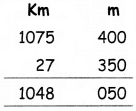Question 2.
250 m 25 cm – 127 m 18 cm
Difference = 250 m 25 cm – 127 m 18 cm
= 123 m 7 cm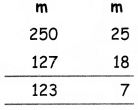Question 3.
27 km 900 m – 18 km 850 m
Difference = 27 km 900 m – 18 km 850 m
= 9 km 50 m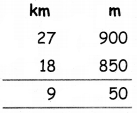Try These (Text Book Page No. 69)

a. 7 m 20 cm × 6
20 cm × 6 = 120 cm
= 1m 20 cm7 m 20 cm × 6 = 43 m 20 cm

b. 15 m 75 cm × 5
75 cm × 5 = 375 cm
= 3m 75 cm15 m 75 cm × 5 = 78 m 75 cm

c. 15 km 200 m × 415 km 200 m × 4 = 60 km 800 m

d. 35 km 500 m × 5
500 m × 5 = 2500 m
= 2 km 500 m35 km 500 m × 5 = 177 km 500 mTry These (Text Book Page No. 70)

a. 750 m 45 cm ÷ 5
b. 49 km 630 m ÷ 7
c. 770 km 550 m ÷ 11